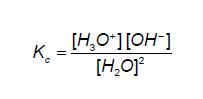# Self-ionisation of Water

For a strong acid, the concentration of the ions will equal the concentration of the acid. e.g. 0.1 M HC1 (aq), [H+] = 0.1 M

A more dilute solution will have a lower [H+]; e.g. 0.001 M HCl (aq), [H+] = 0.001M.

However for a 0.1 M solution of a weak acid such as ethanoic acid, we will find that [H+] « 0.1M. A more dilute solution of ethanoic acid will have an even lower [H+].

Self-ionisation of water:

The amphoteric nature of water is seen even in pure water. Occasionally, one water molecule can donate a proton to another, forming ions.

H2O (I) + H2O (aq) ↔ H3O + (aq) + OH- (aq)

An equilibrium constant Kc expression can be written for this dissociation:The concentration of the ions is extremely small, so the concentration of H2O remains essentially constant. Rearranging the above equation gives:

[H2O]2 KC = [H3O+] [OH-]